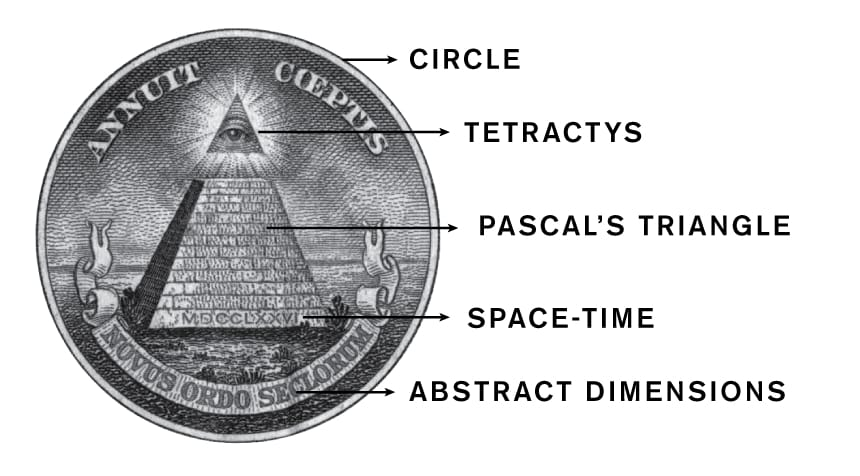# Tetractys, Pascal's Triangle, and Binomial Expansion

It is known that in Ancient Greece, a famous metaphysicist named Pythagoras worshipped a particular shape called the Tetractys. He and his followers believed it to symbolize everything that is perfect about the Universe.

The Tetractys is an equilateral triangle (60°-60°-60°) with 10 interconnected points across exactly four layers. The Pythagoreans believed that this basic shape of nature reveals doorways to the most profound mathematical principles of the Universe.

One of these doorways leads to Pascal’s Triangle.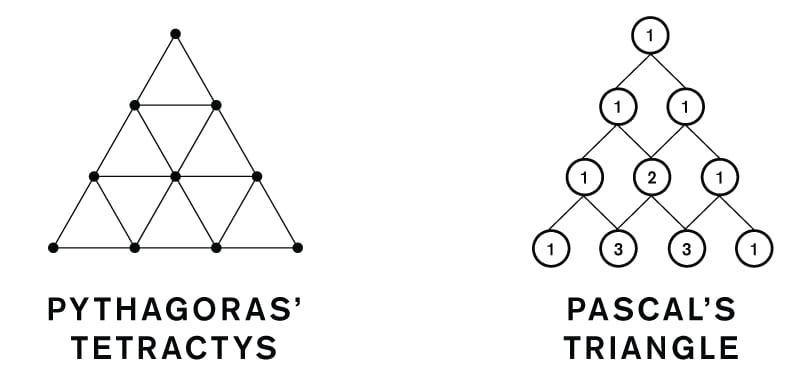Pascal’s triangle is also an ancient concept that Pascal called the ‘Arithmetic Triangle’ when he scientifically re-introduced it in the 17th century. Pascal's triangle is a triangular array of the binomial coefficients, and its form can be considered the base of probability theory while exhibiting an exquisite relationship with the Fibonacci numbers and Phi.

I’ve found that Pascal’s triangle is a special tool used to calculate a very basic concept… a hierarchical pattern…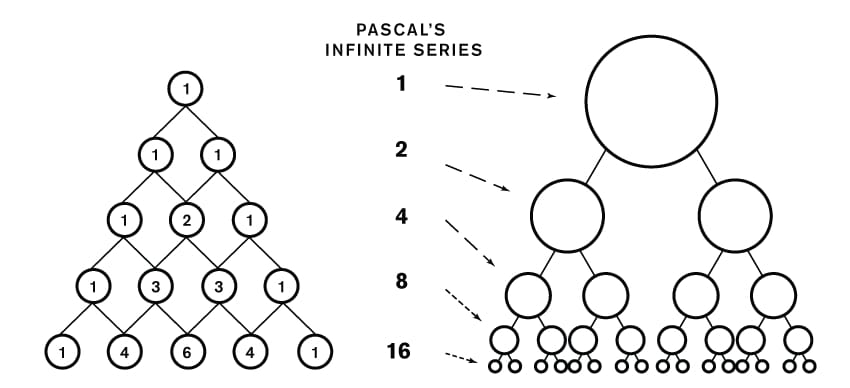The ‘binomial’ coefficients within the Arithmetic Triangle represent the branches per row in the hierarchy.

Pascals triangle compresses 2n circles into just n circles.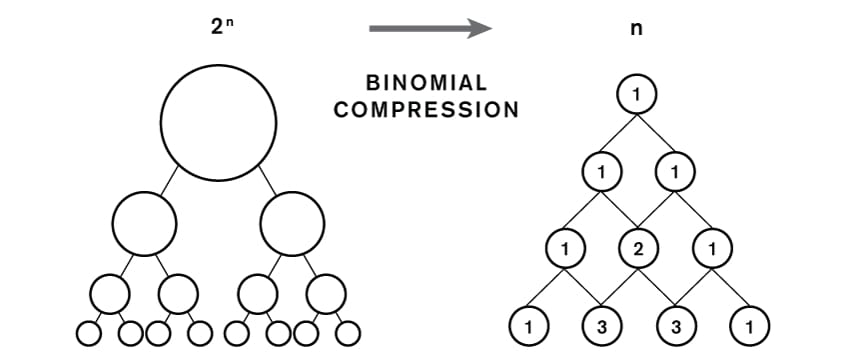The Arithmetic Triangle is nature’s compression algorithm...

When mathematicians employ the binomial expansion (ie. Pascal’s triangle), they are calculating individual branches within a hierarchical pattern (ie. binary tree).

And the Pythagoreans understood this.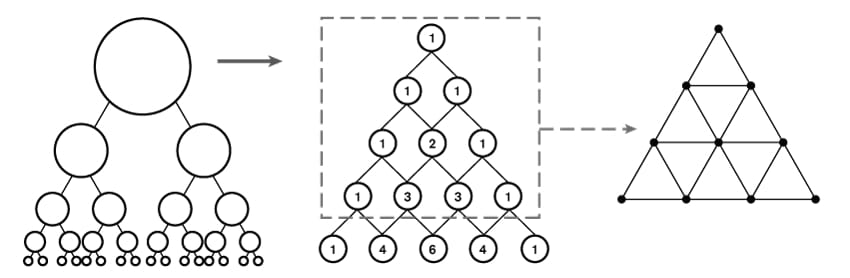Their Tetractys could be thought of as the first few layers, like a capstone, of each hierarchical pattern.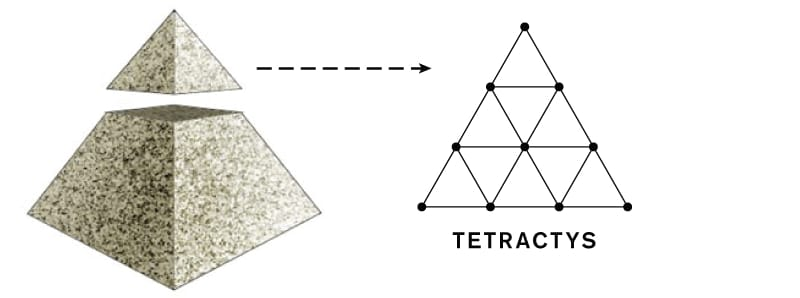It's also where the symbol for the eye of God originates: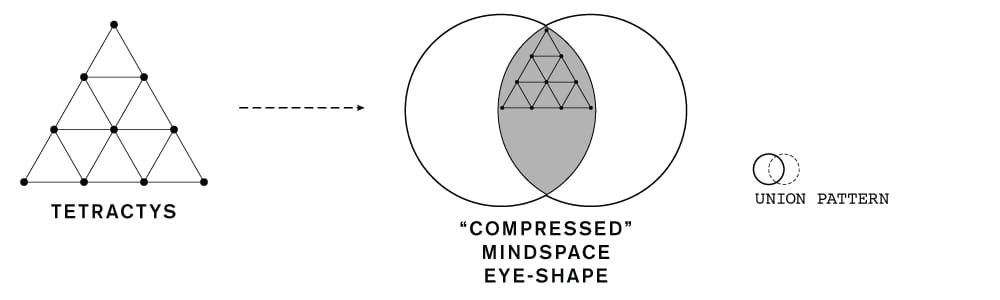So what else does the Tetractys symbolize? Lots of concepts: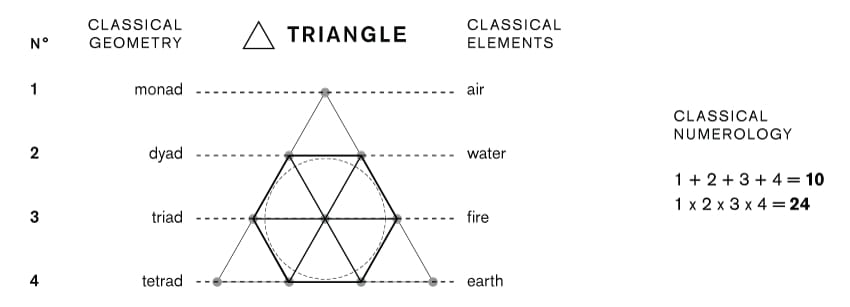But this one is the most exciting: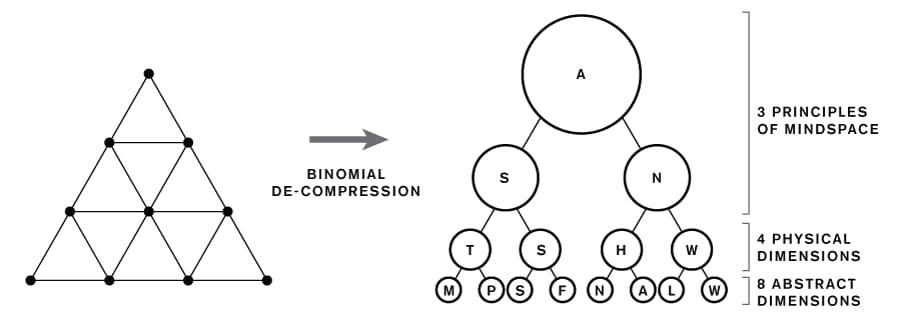The hierarchical pattern that unfolds from the Tetractys reveals the entire configuration of mindspace!

The top two rows represent the circle: the border (A), inside (S), and outside (N). And the bottom two rows are all the dimensions necessary to meaningfully describe it as both a stationary and rotating container.

It also appears that the duality of rest vs. motion are responsible for assembling the hierarchical pattern in the first place - and reveal the concepts of probability and expansion during the process of creating 'depth'.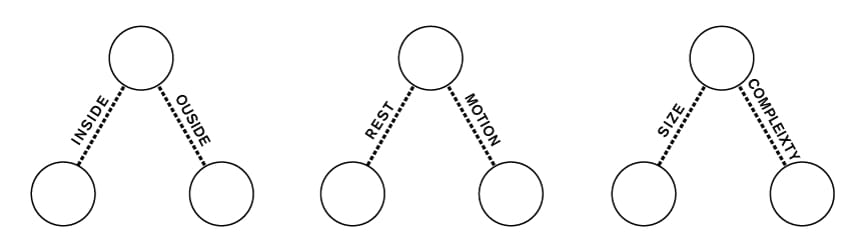So the Tetractys DEEPLY represents the circle, the monad, and the primordial symbol for God - while also being the dimensional framework for mindspace.

And if you think I’m just making up these connections - consider that the \$1 US bill is strikingly designed with this exact symbolism in mind! ...I believe that the founding of the United States upon these symbols, is the source of its global superpower status.